# Simulation of Solid-Liquid Phase Transition Process in Aluminum Foams Using the Lattice Boltzmann Method

Simulation of Solid-Liquid Phase Transition Process in Aluminum Foams Using the Lattice Boltzmann Method

Xinpeng Huang Zhenqian Chen* Juan Shi

School of Energy and Environment, Southeast University, Nanjing 210096, P. R. China

Jiangsu Provincial Key Laboratory of Solar Energy Technology, Nanjing 210096, P. R. China

Corresponding Author Email:
zqchen@seu.edu.cn
Page:
694-700
|
DOI:
https://doi.org/10.18280/ijht.340420
|
Accepted:
|
Published:
31 December 2016
| Citation

OPEN ACCESS

Abstract:

This paper aims to demonstrate the thermodynamics of a thermal storage system based on the latent heat of a composite Phase Change Material (PCM). The paper investigates the flow and heat transfer of solid-liquid phase transitions at the pore level using the Lattice Boltzmann method. The phase transitions process is described using the enthalpy method. A porous medium at the pore scale is constructed here by binarization processing of photograph. The single phase change process is compared with phase transitions in porous media. The simulation results indicate that during the whole heat transfer process, the conduction effect dominates in porous medium composite phase materials while the convection effect has a remarkable influence on a single phase change process. A temperature difference is found between the aluminum foams and phase change materials. Phase transitions in pored aluminum with different porosity are also simulated to validate the feasibility of the enthalpy model at the pore scale.

Keywords:

Aluminum foams, Lattice Boltzmann method, Phase transition, Pore level.

1. Introduction

In recent years, foam metal has enjoyed extensive application prospects in the field of enhanced heat transfer. Since foam metal has a relatively large specific surface area and a complex three dimensional hole mesh structure, it has an efficient heat transfer effect when fluid goes through it. Figure 1 illustrates the physical view of a foam metal ball and its SEM cross-sectional view. At the same time, phase change materials like paraffin and ice-water are used in thermal storage and cool storage engineering projects. Foam metals filled with phase change materials not only have a good heat transfer effect, but also a high energy storage density. Therefore, the porous medium composite phase change materials have a more important research value. They have wider application fields than foam metal.

## 1.png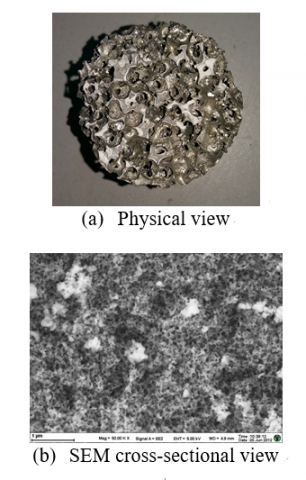Figure 1. Common foam metal ball

Shigeo Kimura and others have studied the saturated porous square section which is heated from below and cooled at the top and has adiabatic side boundaries . M.S. Malashetty, I.S. Shivakumara and Sridhar Kulkami have also studied the same using an analytical method . Shankar Krishnan, Jayathi Y. Murthy and Suresh V. Garimella have used two temperature models to study the heat transfer characteristics of water flow in foam metal by numerical analysis, and have noted the influence of characteristic numbers Ra and Da on the flow and heat transfer . Meanwhile, Nawaf H. Saeid and I. Pop have studied the steady natural convection in square cavities filled with porous medium. They have pointed out that the effect of viscous dissipation slows the rate of heat transfer and as the value of the viscous dissipation effect increases, the average Nu number in porous cavities decreases . Mylene Deleglise, Christophe Binetruy, Philippe Castaing and others have conducted experiments on temperature distribution of the isothermal flow in porous medium 5. Andrew M. Hayes, Jamil A. Khan, Aly H. Shaaban and others have used the two-dimensional numerical calculation model and FluentTM software to study the convection heat transfer problem in a rectanglular heat exchanger. They have also used non-equilibrium heat transfer models of porous media which have been verified by experiments 6. Ibraheem Nasser and H.M. Duwairi have conducted research on convection heat transfer in porous media 7. Mohamed Bachiri and Ahcene Bouabdallah have studied the natural convection theoretically by the direct integration of momentum and energy equations 8. Halima Usman and others have investigated heat and mass transfer flow along a vertical channel in a porous medium with a radiation effect 9. Chen Yuan-Yuan, Li Ben-Wen and Zhang Jing-Kui have used the spectral collocation method to study the heat transfer problem of natural convection in square cavities filled with porous media. They have established the partial equilibrium model and the local non-equilibrium model of this problem. Their results indicate that the method is highly consistent with the published conclusions on the problem 10.

The research above is about heat transfer and flow in porous media. In addition, other scholars have studied the phase transitions and multi-phases flow. Qingni Yuan, Qingyun Yuan and Feilong Du have studied the two-phase fluid in fried pepper sauce using CFD tools 11. Finally, there are experimental studies by other scholars on the heat transfer performance of foamed metal used in heat exchangers 12-15.

Most of the current work was done on the assumption of the local thermal equilibrium. It is not hard to understand why local thermal equilibrium was used. To simulate the convective heat transfer process in metal foam through  the macroscopic method is difficult in mathematical processing, as th Navier-Stokes equation needs to be decoupled. However, the local thermal equilibrium is somewhat unrealistic.

This paper shows the temperature differentials between the PCM and metal foam under local thermal non-equilibrium conditions. The author has used a mesoscopic method (the Lattice-Boltzmann method) to solve the Navier-Stokes equation. The mathematical processing was concise and clear as shown below. Adopting Boltzmann’s collision-migration model, the Lattice- Boltzmann method has special advantages in handling the mazy boundary inside the porous foamed metal. Besides, the single phase change process is also analyzed in this paper and compared with phase transitions in porous media.

2. Mathematical Model

A sketch map of the composite phase change material compounded with porous medium is shown in Figure 2. Amorphous material paraffin is filled in foamed aluminum to form the composite phase change material. The side of the foam metal skeleton square enclosure is H, where the statistical average pore diameter is l. The left and right sides are held at constant temperatures T1 and T2. The top and bottom sides are adiabatic. The paraffin and aluminum foam are at equilibrium initially, and at temperature T2. When t>0, the temperature of the left side is raised to T1. The assumption below highlight the key point of the discussion:

1) Ignore paraffin volume change before and after phase change,

2) The thermophysical property of paraffin in the solid and liquid zones remains unchanged,

3) The Bounssinesq approximation is only invoked in the fluid phase.

## 2.png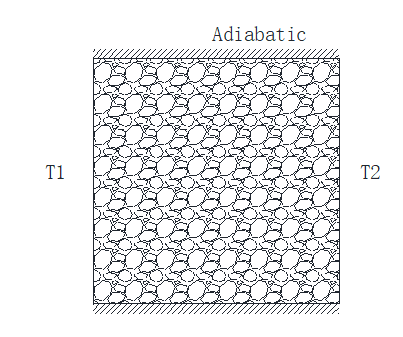Figure 2. Sketch map of foamed aluminum filled with paraffin

Based on the assumption above, the governing equations of flow and heat transfer are:

$\frac{\partial \rho u}{\partial x}+\frac{\partial \rho v}{\partial y}=0$(1)

$\frac{\partial \rho u}{\partial t}+\frac{\partial(\rho u u)}{\partial x}+\frac{\partial(\rho u v)}{\partial y}=-\frac{\partial p}{\partial x}+\frac{\partial}{\partial x}\left(\mu \frac{\partial u}{\partial x}\right)+\frac{\partial}{\partial y}\left(\mu \frac{\partial u}{\partial y}\right)+f_{x}$(2)

$\frac{\partial \rho v}{\partial t}+\frac{\partial(\rho u v)}{\partial x}+\frac{\partial(\rho v v)}{\partial y}=-\frac{\partial p}{\partial y}+\frac{\partial}{\partial x}\left(\mu \frac{\partial v}{\partial x}\right)+\frac{\partial}{\partial y}\left(\mu \frac{\partial v}{\partial y}\right)$$+f_{y}+\rho_{0} g \beta T^{\prime}$(3)

$\frac{\partial \rho c T}{\partial t}+\frac{\partial(\rho c u T)}{\partial x}+\frac{\partial(\rho c v T)}{\partial y}=\frac{\partial}{\partial x}\left(k \frac{\partial T}{\partial x}\right)+\frac{\partial}{\partial y}\left(k \frac{\partial T}{\partial y}\right)$(4)

Regarding the four equations above, Eq(1) is the mass conservation equation; Eq(2) and Eq(3) are momentum conservation equations in two-dimensional scale; Eq(4) is the energy conservation equation. In Eq(2) and Eq(3), f is the volume force generated from the porous medium.

To generalize the solving of the problem, dimensionless processing was done on the equation set above. Let H be the characteristic length. Let H2 be the characteristic time. Let ν/H be the characteristic velocity. Let T1 and T2 be the characteristic temperatures, then we can deduce the dimensionless temperature expressed by θ = (T-T2)/(T1-T2). As a result, the dimensionless equation set can be expressed as follows:

$\frac{\partial U}{\partial X}+\frac{\partial V}{\partial Y}=0$(5)

$\frac{\partial U}{\partial T}+\frac{\partial(U U)}{\partial X}+\frac{\partial(U V)}{\partial Y}=-\frac{\partial P}{\partial X}+\frac{\partial^{2} U}{\partial X^{2}}+\frac{\partial^{2} U}{\partial Y^{2}}+F_{x}$(6)

$\frac{\partial V}{\partial T}+\frac{\partial(U V)}{\partial X}+\frac{\partial(V V)}{\partial Y}=-\frac{\partial P}{\partial Y}+\frac{\partial^{2} V}{\partial X^{2}}+\frac{\partial^{2} V}{\partial Y^{2}}+F_{y}+R a \theta$(7)

$\frac{\partial \theta}{\partial T}+\frac{\partial(U \theta)}{\partial X}+\frac{\partial(V \theta)}{\partial Y}=\frac{1}{R e P_{T}}\left(\frac{\partial^{2} \theta}{\partial X^{2}}+\frac{\partial^{2} \theta}{\partial Y^{2}}\right)$(8)

where characteristic numbers Ra=gβT’H3/ (αν), Re=uH/ν, Pr=ν/α.

For porous media flow problems of pore size, the LBE evolution equation is expressed as:

$f_{k}(x+\Delta x, t+\Delta t)=f_{k}(x, t)\left(1-\omega_{m}\right)+\omega_{m} f_{k}^{e q}(x, t)+S_{f}$(9)

where the equilibrium distribution function is expressed as:

$f_{k}^{e q}=w_{k} \Phi(x, t)\left(1+\frac{c_{k} \vec{u}}{c_{s}^{2}}\right)$(10)

in Eq (9), $\omega_{m}=\frac{1}{3 v+0.5}$.

For the flow problem of heat lattice in porous media, Guo Zhaoli has put forward a solution of two distribution functions 16. Through adding a temperature distribution function similar to the speed distribution function, the problem can be solved.

$g_{k}(x+\Delta x, t+\Delta t)=g_{k}(x, t)\left(1-\omega_{n}\right)+\omega_{n} g_{k}^{e q}(x, t)$(11)

where the equilibrium distribution function of temperature is expressed as:

$g_{k}^{e q}=w_{k} \Phi(x, t)\left(1+\frac{c_{k} \vec{u}}{c_{s}^{2}}\right)$(12)

in Eq (11), $\omega_{n}=\frac{1}{3 \alpha+0.5}$.

For the solid-liquid phase transitions problem, temperature and the solid-liquid ratio is coupled with each other. Then the enthalpy method was used to deal with this problem 17. At time step n and iteration k, the macroscopic temperature is calculated:

$T^{n, k}=\sum_{i} g_{i}^{n, k}$(13)

The local enthalpy is obtained by:

$E n^{n, k}=c T^{n, k}+\gamma_{1}^{n, k-1} L_{f}$(14)

with the solid-liquid ratio γ of the previous iteration. Finally the enthalpy method is used to linearly interpolate the melt fraction:

$\gamma_{f}^{n, k}=\left\{\begin{array}{cc}{0} & {E n^{n, k}<E n_{s}=c T_{m}} \\ {\frac{E n^{n, k}+E n_{s}}{E n_{l}-E n_{s}}} & {E n_{s} \leq E n^{n, k} \leq E n_{s}+L_{f}} \\ {1} & {E n^{n, k}>E n_{s}+L_{f}}\end{array}\right.$(15)

The collision is then calculated until the temperature and the melt fraction field converge to within a set tolerance.

For the problem of porous medium reconstruction at the pore scale, binarization processing of a photograph was used. In Figure 1, the porous medium can be characterized by the function δ(x, y), which can be expressed by:

$\delta(x, y)=\left\{\begin{array}{lr}{0} & {\text { skeleton }} \\ {1} & {\text { pore }}\end{array}\right.$(16)

After the photograph is processed, Figure 1 is translated into Figure 3.

## 3.png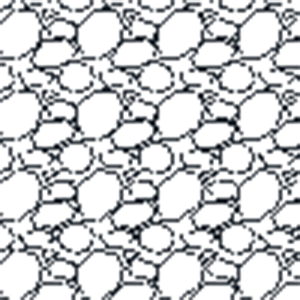Figure 3. Sketch map of foamed aluminum after photograph processing

In the calculation, set Ra=5×105, Pr=5.0 and a dimensionless temperature difference between the left side and the right side of T’=1.0, use the 100×100 mesh grid and the D2Q9 model. The calculation process is shown below:

## 4.png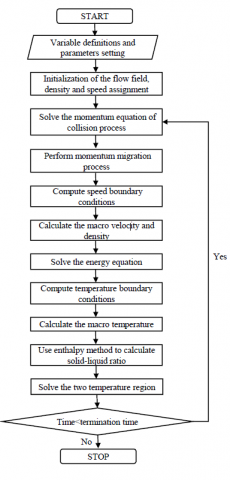Figure 4. Flow chart of calculation

3. Results and Discussion

First, sketch maps with different porosities are compared in Figure 5. Figure 5(a) is pure Phase Change Material (PCM) after binarization processing, Figure 5(b) is a Lena image after binarization processing, and Figure 5(c) is Figure 3 after binarization processing.

## 5a.png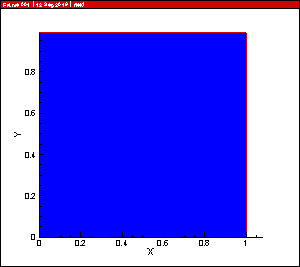(a) Pure PCM

## 5b.png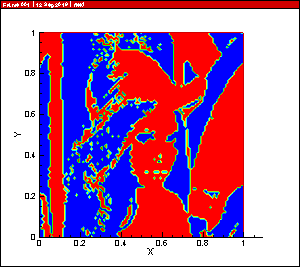(b) Foamed metal ( porosity=0.522)

## 5c.png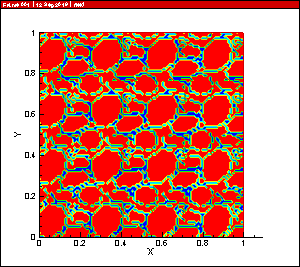(c) Foamed metal ( porosity=0.788)

Figure 5. Comparison of sketch maps used to calculate

Now the foamed metal with a porosity of 0.778 is the same as in Figure 3. We calculated the temperature fields of the three sketch maps and the results are shown in Figures 6 - 9.

## 6a.png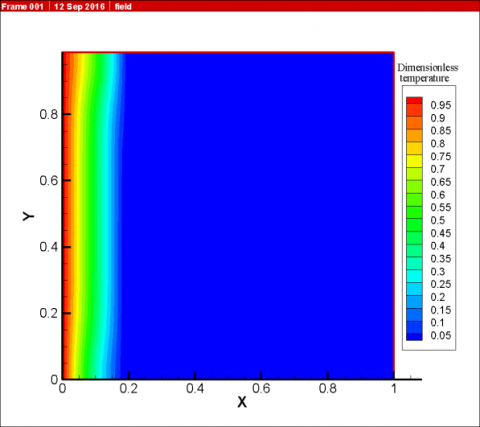(a) After calculating 1,000 steps

## 6b.png(b) After calculating 10,000 steps

## 6c.png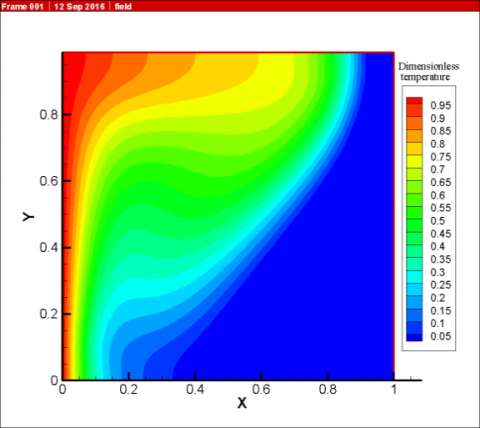(c) After calculating 40,000 steps

## 6d.png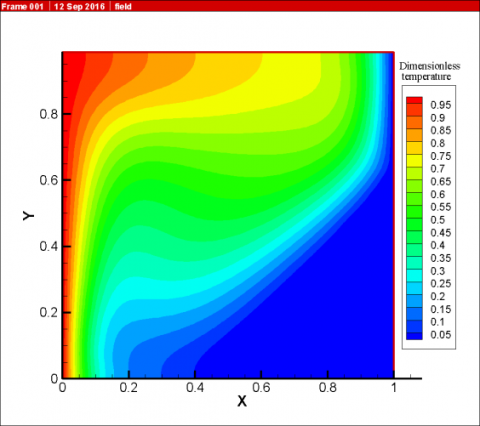(d) After calculating 50,000 steps

Figure 6. Snapshots of the pure PCM temperature field in different dimensionless times

## 7a.png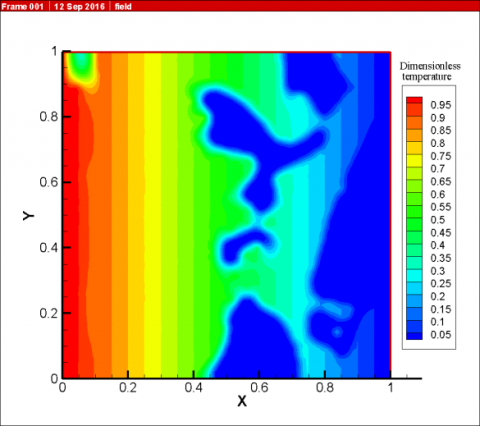(a) After calculating 1,000 steps

## 7b.png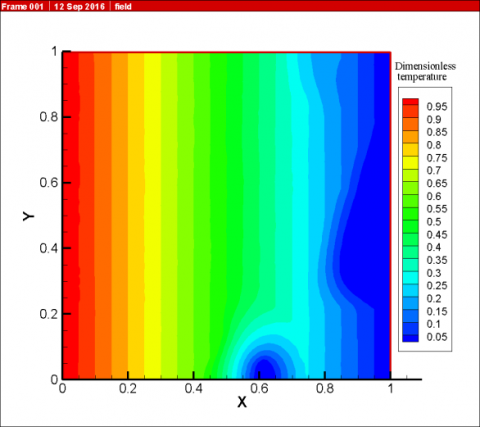(b) After calculating 10,000 steps

## 7c.png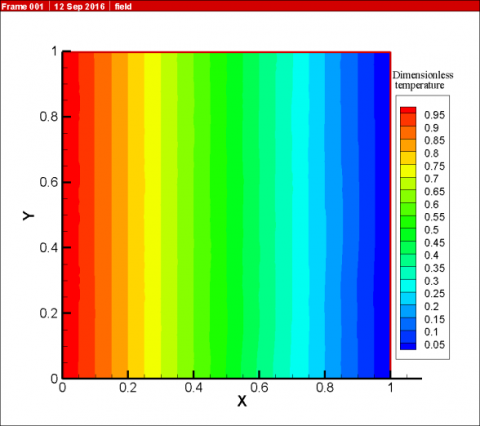(c) After calculating 40,000 steps

## 7d.png(d) After calculating 50,000 steps

Figure 7. Snapshots of the sketch (porosity=0.522) temperature field in different dimensionless times

## 8a.png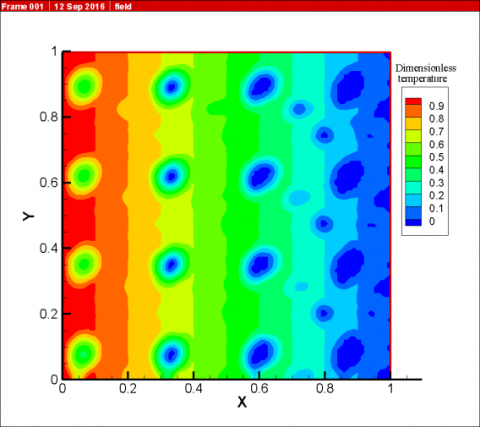(a) After calculating 1,000 steps

## 8b.png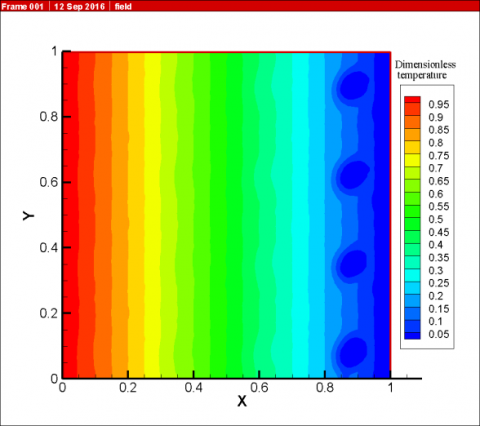(b) After calculating 10,000 steps

## 8c.png(c) After calculating 40,000 steps

## 8d.png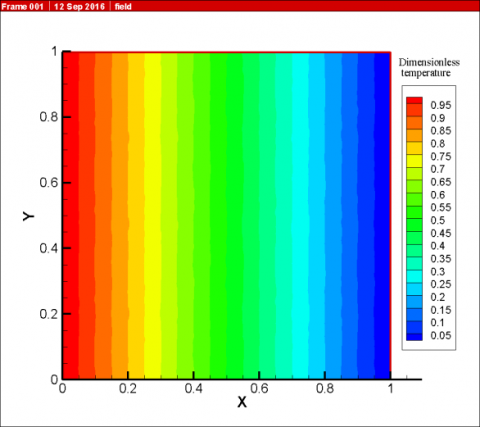(d) After calculating 50,000 steps

Figure 8. Snapshots of the sketch (porosity=0.778) temperature field in different dimensionless times

## 9.png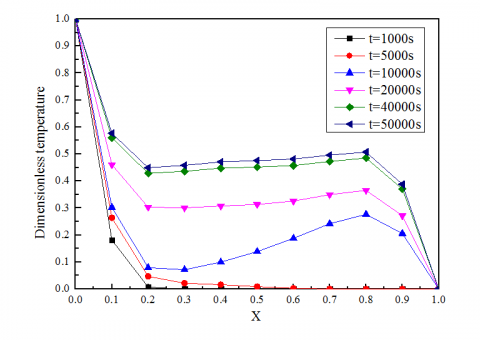Figure 9. Average temperature variation by time in the center of the sketch (porosity=0.778)

From Figure 6, after calculating 1,000 steps in the initial time, the isotherm distribution is approximating linear near the left side wall of pure PCM. The heat exchange between the left and right side at the initial moment is mainly the effect of heat conduction. As time goes by, the mechanism of heat transfer between the left and right sides gradually changes from heat conduction to heat convection. The calculated results shown in Figure 5 fully agree with the existing experimental results obtained by Zhenqian Chen18.

However, when one compares Figures 7-8 to Figure 6, one can see the heat convection effect is not obvious and the mechanism of heat transfer is dominated by heat conduction all the time. It is also obvious that the migration rate of the temperature field is accelerated as the ratio of foam metal is increased. From Figures 7 (a) and 8 (a), during the transient state, large temperature differences between the foamed aluminum and PCM can be observed. It is also found that the temperature differences were observed by infrared camera in Ref. .

In Figure 9, it can be observed that, under the same simulation conditions, the average temperature increases with the timestep. It reaches a steady heat transfer state when timesteps=50,000.

Lastly, when one compares Figures 7 (d) and 8 (d) to Figure 6 (d), it is verified that compared to single phase change materials, the composite phase change materials significantly improve the performance of the thermal conductivity of materials. The microscope image capture comparing the melting interface shapes of the pure paraffin and paraffin/foam composite confirms the simulation results18.

4. Conclusions

The Lattice Boltzmann method has been successfully used to analyze the melting process of composite phase change materials at the pore scale. The enthalpy method has been applied and the local non-equilibrium condition considered. The results show that in the unsteady-state of heat transfer, the temperature differences between metal foam and PCM cannot be ignored, while in the steady-state temperature differences are small. Moreover, the results show that the heat transfer mechanism in the pure PCM is dominated by heat convection, while in the composite PCM the heat transfer mechanism is dominated by heat conduction. In sum, the results fully agree with the existing experimental observations.

Acknowledgment

The authors gratefully acknowledge the supports of National Natural Science Foundation of China (Grant No.50776015) and National Natural Science Foundation of China (Grant No.51606037).

Nomenclature
 c specific heat, J. kg-1. K-1 En local enthalpy, J. kg-1 F dimensionless additional force length f additional force, kg.m.s-2 g local acceleration of gravity, m.s-2 k thermal conductivity, W.m-1.K-1 L latent heat, J.kg-1 P dimensionless pressure length p pressure, kg.m-1.s-2 T tempreture, K t time, s U dimensionless velosity length in x direction u velosity in the x direction, m.s-1 V dimensionless velosity length in y direction v velosity in the y direction, m.s-1 w weight coefficient in LBM method Ra Rayleigh number Re local Reynolds number Pr local Prandtl number Greek symbols α thermal diffusivity, m2. s-1 β thermal expansion coefficient, K-1 γ solid-liquid ratio, dimensionless δ Dirac function Ɵ dimensionless temperature µ dynamic viscosity, kg. m-1.s-1 ρ density, kg.m-3 ν kinematic viscosity, m2.s-1 $\phi$ distribution function ω relaxation frequency, s-1 Subscripts f external force item k the motion direction of lattice, in D2Q9 model k=9 l liquid zone m momentum n heat diffusion s solid zone
References

 Shigeo Kimura, Naotaka Ishikawa, Nobuyoshi Komatsu, “On realizable convection patterns in a saturated porous square section,” International Journal of Heat and Technology, vol. 34, Special Issue 1, pp. S91-S94, 2016. DOI: 10.18280/ijht.34S111.

 M.S.Malashetty, I.S.Shivakumara, Sridhar Kulkarni, “The onset of Lapwood–Brinkman convection using a thermal non-equilibrium model,” International Journal of Heat and Mass Transfer., vol. 48, pp. 1155-1163, 2005. DOI: 10.1016/j.ijheatmasstransfer.2004.09.027.

 Shankar Krishnan, Jayathi Y. Murthy, Suresh V. Garimella, “A two-temperature model for the analysis of passive thermal control systems,” Transactions of the ASME, vol. 126, pp. 628-637, 2004. DOI: 10.1115/1.1773194.

 Nawaf H.Saeid, I.Pop, “Viscous dissipation effects on free convection in a porous cavity,” Int. Comm. Heat Mass Transfer, vol. 31, no. 5, pp. 723-7, 2016. DOI: 10.1016/S0735-1933(04)00059-4.

 Mylene Deleglise, Christophe Binetruy, Philippe Castaing, Patricia Krawczak, “Use of non-local equilibrium theory to predict transient temperature during non-isothermal resin flow in a fibrous medium,” International Journal of Heat and Mass Transfer, vol. 50, pp. 2317-2324, 2007. DOI: 10.1016/j.ijheatmasstransfer.2006.10.020.

 Andrew M. Hayes, Jamil A. Khan, Aly H. Shaaban, Ian G.Spearing, “The thermal modeling of a matrix heat exchanger using a porous medium and the thermal non-equilibrium model,”     International Journal of Thermal Sciences, vol. 47, no. 10, pp. 1306-1315, 2008. DOI: 10.1016/j.ijthermalsci.2007.11.005.

 Ibraheem Nasser, H.M.Duwairi, “Thermal dispersion effects on convection heat transfer in porous media with viscous dissipation,” International Journal of Heat and Technology, vol. 34, no. 2, pp. 207-212, 2016. DOI: 10.18280/ijht.340208.

 Mohamed Bachiri, Ahcene Bouabdallah, “Natural convection study by the direct integration of the momentum and energy equations,” International Journal of Heat and Technology, vol. 34, no. 2, pp. 181-185, 2016. DOI: 10.18280/ijht.340204.

 Halima Usman, Fazle Mabood, Giulio Lorenzini, “Heat and mass transfer along vertical channel in porous medium with radiation effect and slip condition,” International Journal of Heat and Technology, vol. 34, no. 1, pp. 129-136, 2016. DOI: 10.18280/ijht.340119.

 Yuan-Yuan Chen, Ben-Wen Li, Jing-Kui Zhang, “Spectral collocation method for natural convection in a square porous cavity with local thermal equilibrium and non-equilibrium models,” International Journal of Heat and Mass Transfer, vol. 96, pp. 84-96, 2016. DOI: 10.1016/j.ijheatmasstransfer.2016.01.007.

 Qingni Yuan, Qingyun Yuan, Feilong Du, “The characteristics research of solid-liquid two-phase fluid in the filling process of fried pepper sauce,” International Journal of Heat and Technology, vol. 34, no. 2, pp. 221-226, 2016. DOI: 10.18280/ijht.340210.

 Zhang Tao, Yu Jianzu, “Experiment of solid-liquid phase change in copper foam,” Journal of Beijing University of Aeronautics and Astronautics, vol. 33, no. 9, pp. 1021-1024, 2007.

 Zhang Tao, Yu Jianzu, “Experiment of solid-liquid phase change in copper foam,” Journal of Refrigeration, vol. 28, no. 6, pp. 13-17, 2007.

 Qiu Haiping, Shi Mingheng, “An experimental investigation on the flow and heat transfer characteristics for aluminum foam,” Journal of Engineering Thermophysics, vol. 26, no. 6, pp. 1016-1018, 2005.

 Wang Xiaolu, Jiang Peixue, Shan Huozhi , “Investigation of convection heat transfer in metal foams and mini-fin structures,” Journal of Engineering Thermophysics, vol. 29, no. 1, pp. 121-123, 2008.

 Guo Z L, Zhao T S, “A lattice Boltzmann model for convection heat transfer in porous media,” Numerical Heat Transfer. Part B, vol. 47, pp. 156-177, 2005.

 DOI: 10.1080/10407790590883405.

 Christian Huber, Andrea Parmigiani, Bastien Chopard, Michael Manga, Olivier Bachmann, “Lattice Boltzmann model for melting with natural convection,” International Journal of Heat and Fluid Flow, vol. 29, no. 5, pp. 1469-1480, 2008. DOI: 10.1016/j.ijheatfluidflow.2008.05.002.

 Zhenqian Chen, Dongyan Gao, Juan Shi, “Experimental and numerical study on melting of phase change materials in metal foams at pore scale,” International Journal of Heat and Mass transfer, vol. 72, pp. 646-655, 2014. DOI: 10.1016/j.ijheatmasstransfer.2014.01.003.